Parabola

For other uses, see Parabola (disambiguation).

A parabola (/pəˈræbələ/; plural parabolas or parabolae, adjective parabolic, from Greek: παραβολή) is a two-dimensional, mirror-symmetrical curve, which is approximately U-shaped when oriented as shown in the diagram below, but which can be in any orientation in its plane. It fits any of several superficially different mathematical descriptions which can all be proved to define curves of exactly the same shape.

One description of a parabola involves a point (the focus) and a line (the directrix). The focus does not lie on the directrix. The parabola is the locus of points in that plane that are equidistant from both the directrix and the focus. Another description of a parabola is as a conic section, created from the intersection of a right circular conical surface and a plane which is parallel to another plane which is tangential to the conical surface.[lower-alpha 1] A third description is algebraic. A parabola is a graph of a quadratic function, y = x2, for example.[lower-alpha 2]

The line perpendicular to the directrix and passing through the focus (that is, the line that splits the parabola through the middle) is called the "axis of symmetry". The point on the parabola that intersects the axis of symmetry is called the "vertex", and is the point where the parabola is most sharply curved. The distance between the vertex and the focus, measured along the axis of symmetry, is the "focal length". The "latus rectum" is the chord of the parabola which is parallel to the directrix and passes through the focus. Parabolas can open up, down, left, right, or in some other arbitrary direction. Any parabola can be repositioned and rescaled to fit exactly on any other parabola — that is, all parabolas are geometrically similar.

Parabolas have the property that, if they are made of material that reflects light, then light which travels parallel to the axis of symmetry of a parabola and strikes its concave side is reflected to its focus, regardless of where on the parabola the reflection occurs. Conversely, light that originates from a point source at the focus is reflected into a parallel ("collimated") beam, leaving the parabola parallel to the axis of symmetry. The same effects occur with sound and other forms of energy. This reflective property is the basis of many practical uses of parabolas.

The parabola has many important applications, from a parabolic antenna or parabolic microphone to automobile headlight reflectors to the design of ballistic missiles. They are frequently used in physics, engineering, and many other areas.

Strictly, the adjective parabolic should be applied only to things that are shaped as a parabola, which is a two-dimensional shape. However, as shown in the last paragraph, the same adjective is commonly used for three-dimensional objects, such as parabolic reflectors, which are really paraboloids. Sometimes, the noun parabola is also used to refer to these objects. Though not perfectly correct, this usage is generally understood.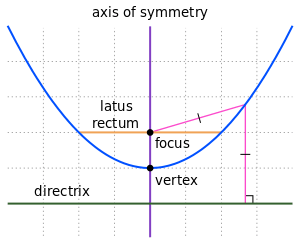Part of a parabola (blue), with various features (other colours). The complete parabola has no endpoints. In this orientation, it extends infinitely to the left, right, and upward.

History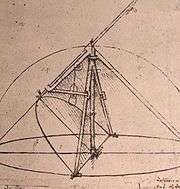Parabolic compass designed by Leonardo da Vinci

The earliest known work on conic sections was by Menaechmus in the fourth century BC. He discovered a way to solve the problem of doubling the cube using parabolas. (The solution, however, does not meet the requirements of compass-and-straightedge construction.) The area enclosed by a parabola and a line segment, the so-called "parabola segment", was computed by Archimedes via the method of exhaustion in the third century BC, in his The Quadrature of the Parabola. The name "parabola" is due to Apollonius who discovered many properties of conic sections. It means "application", referring to "application of areas" concept, that has a connection with this curve, as Apollonius had proved. The focus–directrix property of the parabola and other conics is due to Pappus.

Galileo showed that the path of a projectile follows a parabola, a consequence of uniform acceleration due to gravity.

The idea that a parabolic reflector could produce an image was already well known before the invention of the reflecting telescope. Designs were proposed in the early to mid seventeenth century by many mathematicians including René Descartes, Marin Mersenne, and James Gregory. When Isaac Newton built the first reflecting telescope in 1668, he skipped using a parabolic mirror because of the difficulty of fabrication, opting for a spherical mirror. Parabolic mirrors are used in most modern reflecting telescopes and in satellite dishes and radar receivers.

Equation in Cartesian coordinates

Let the directrix be the line x = −p and let the focus be the point (p, 0). If (x, y) is a point on the parabola then, by definition of a parabola, it is the same distance from the directrix as the focus; in other words:Squaring both sides and simplifying producesas the equation of the parabola. By interchanging the roles of x and y one obtains the corresponding equation of a parabola with a vertical axis asThe equation can be generalized to allow the vertex to be at a point other than the origin by defining the vertex as the point (h, k). The equation of a parabola with a vertical axis then becomesThe last equation can be rewrittenso the graph of any function which is a polynomial of degree 2 in x is a parabola with a vertical axis.

More generally, a parabola is a curve in the Cartesian plane defined by an irreducible equation — one that does not factor as a product of two not necessarily distinct linear equations — of the general conic formwith the parabola restriction thatwhere all of the coefficients are real and where A and C are not both zero. The equation is irreducible if and only if the determinant of the 3 × 3 matrixis non-zero: that is, if ACB2/4F + BED/4CD2/4AE2/4 ≠ 0. The reducible case, also called the degenerate case, gives a pair of parallel lines, possibly real, possibly imaginary, and possibly coinciding with each other.

Diagram, description, and definitions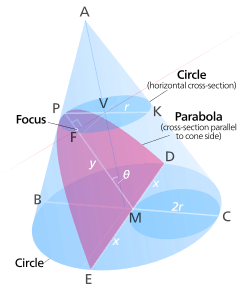Cone with cross-sections (To enlarge, click on diagram. To shrink, go to previous page.)

The diagram represents a cone with its axis vertical.[lower-alpha 3] The point A is its apex. An inclined cross-section of the cone, shown in pink, is inclined from the vertical by the same angle, θ, as the side of the cone. According to the definition of a parabola as a conic section, the boundary of this pink cross-section, EPD, is a parabola.

A horizontal cross-section of the cone passes through the vertex, P, of the parabola. This cross-section is circular, but appears elliptical when viewed obliquely, as is shown in the diagram. Its centre is V, and PK is a diameter. We will call its radius r.

Another horizontal, circular cross-section of the cone is farther from the apex, A, than the one just described. It has a chord DE, which joins the points where the parabola intersects the circle. Another chord, BC, is the perpendicular bisector of DE, and is consequently a diameter of the circle. These two chords and the parabola's axis of symmetry, PM, all intersect at the point M.

All the labelled points, except D and E, are coplanar. They are in the plane of symmetry of the whole figure. This includes the point F, which is not mentioned above. It is defined and discussed below, in the paragraph "Position of the focus".

Let us call the length of DM and of EM x, and the length of PM y.

The lengths of BM and CM are:(triangle BPM is isosceles.)(PMCK is a parallelogram.)

Using the intersecting chords theorem on the chords BC and DE, we get:Substituting:Rearranging:For any given cone and parabola, r and θ are constants, but x and y are variables which depend on the arbitrary height at which the horizontal cross-section BECD is made. This last equation shows the relationship between these variables. They can be interpreted as Cartesian coordinates of the points D and E, in a system in the pink plane with P as its origin. Since x is squared in the equation, the fact that D and E are on opposite sides of the y-axis is unimportant. If the horizontal cross-section moves up or down, toward or away from the apex of the cone, D and E move along the parabola, always maintaining the relationship between x and y shown in the equation. The parabolic curve is therefore the locus of points where the equation is satisfied, which makes it a Cartesian graph of the quadratic function in the equation.

This discussion started from the definition of a parabola as a conic section, but it has now led to a description as a graph of a quadratic function. This shows that these two descriptions are equivalent. They both define curves of exactly the same shape.

Focal length

It is proved in the preceding section[lower-alpha 4] that if a parabola has its vertex at the origin, and if it opens in the positive y direction, then its equation is y = x2/4f, where f is its focal length.[lower-alpha 5] Comparing this with the last equation above shows that the focal length of the parabola in the cone is r sin θ.

Position of the focus

In the diagram above, the point V is the foot of the perpendicular from the vertex of the parabola to the axis of the cone. The point F is the foot of the perpendicular from the point V to the plane of the parabola.[lower-alpha 6] By symmetry, F is on the axis of symmetry of the parabola. Angle VPF is complementary to θ, and angle PVF is complementary to angle VPF, therefore angle PVF is θ. Since the length of PV is r, the distance of F from the vertex of the parabola is r sin θ. It is shown above that this distance equals the focal length of the parabola, which is the distance from the vertex to the focus. The focus and the point F are therefore equally distant from the vertex, along the same line, which implies that they are the same point. Therefore, the point F, defined above, is the focus of the parabola.

Other geometric definitions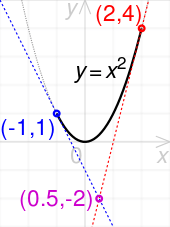A parabolic segment can be constructed exactly from a quadratic Bézier curve: tangents to the parabola at the curve's end points intersect at its control point

A parabola may also be characterized as a conic section with an eccentricity of 1. As a consequence of this, all parabolas are similar, meaning that while they can be different sizes, they are all the same shape. Another consequence is that the universal parabolic constant is the same for all parabolas. A parabola can also be obtained as the limit of the shape of an ellipse where one focus is kept fixed as the other is allowed to move arbitrarily far away in one direction. In this sense, a parabola may be considered an ellipse that has one focus at infinity. The parabola is an inverse transform of a cardioid.

A parabola has a single axis of reflective symmetry, which passes through its focus and is perpendicular to its directrix. The point of intersection of this axis and the parabola is called the vertex. A parabola spun about this axis in three dimensions traces out a shape known as a paraboloid of revolution.

The parabola is found in numerous situations in the physical world (see below).

Equations

Cartesian

In the following equations h and k are the coordinates of the vertex (h, k) of the parabola and p is the distance from the vertex to the focus and the vertex to the directrix.

Vertical axis of symmetrywhereParametric form:Horizontal axis of symmetrywhereParametric form:General parabola

The general form for a parabola isThis result is derived from the general conic equation given below:and the fact that, for a parabola,.

The equation for a general parabola with a focus point F(u, v), and a directrix in the formisLatus rectum, semilatus rectum, and polar coordinates

In the polar coordinate system, a parabola with the focus at the origin and the directrix parallel to the y-axis, and opening to the left, is given by the equationwhere l is the semilatus rectum: the distance from the focus to the parabola itself, measured along a line perpendicular to the axis of symmetry. Note that this equals the perpendicular distance from the focus to the directrix, and is twice the focal length, which is the distance from the focus to the vertex of the parabola.

The latus rectum is the chord that passes through the focus and is perpendicular to the axis of symmetry. It has a length of 2l.

Dimensions of parabolas with axes of symmetry parallel to the y-axis

These parabolas have equations of the form y = ax2 + bx + c. By interchanging x and y the parabolas' axes of symmetry become parallel to the x-axis.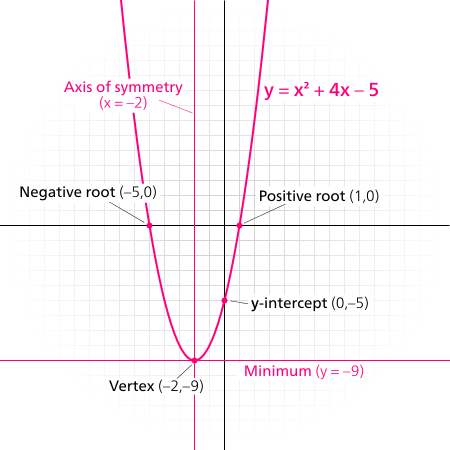Some features of a parabola

Coordinates of the vertex

The x-coordinate at the vertex can be found by completing the square to put the equation y = ax2 + bx + c in vertex form, or by differentiating the original equation, setting the resulting dy/dx = 2ax + b equal to zero (a critical point), and solving for x. Both methods yield x = −b/2a.

Substituting this into the original equation yieldsThese terms can be combined over a common denominator:where D = b2 − 4ac is the discriminant.

Thus, the vertex is at the point (b/2a, −D/4a).

Coordinates of the focus

Since the axis of symmetry of this parabola is parallel with the y-axis, the x-coordinates of the focus and the vertex are equal. The coordinates of the vertex are calculated in the preceding section. The x-coordinate of the focus is therefore also b/2a.

To find the y-coordinate of the focus, consider the point, P, located on the parabola where the slope is 1, so the tangent to the parabola at P is inclined at 45 degrees to the axis of symmetry. Using the reflective property of a parabola, we know that light which is initially travelling parallel to the axis of symmetry is reflected at P toward the focus. The 45-degree inclination causes the light to be turned 90 degrees by the reflection, so it travels from P to the focus along a line that is perpendicular to the axis of symmetry and to the y-axis. This means that the y-coordinate of P must equal that of the focus.

By differentiating the equation of the parabola and setting the slope to 1, we find the x-coordinate of P:Substituting this value of x in the equation of the parabola, we find the y-coordinate of P, and also of the focus:where D = b2 − 4ac is the discriminant, as used in the Coordinates of the vertex section.

The focus is therefore the pointAxis of symmetry, focal length, latus rectum, and directrix

The above coordinates of the focus of a parabola of the formcan be compared with the coordinates of its vertex, which are derived in the section Coordinates of the vertex, above, and arewhere D = b2 − 4ac.

The axis of symmetry is the line which passes through both the focus and the vertex. In this case, it is vertical, with equation.

The focal length of the parabola is the difference between the y-coordinates of the focus and the vertex:It is sometimes useful to invert this equation and use it in the form a = 1/4f. See the section Conic section and quadratic form, above.

The point where the slope of the parabola is 1 lies at one end of the latus rectum. The length of the semilatus rectum (half of the latus rectum) is the difference between the x-coordinates of this point, which is considered as P in the above derivation of the coordinates of the focus, and of the focus itself. Thus, the length of the semilatus rectum iswhere f is the focal length.

The total length of the latus rectum is therefore four times the focal length.

Measured along the axis of symmetry, the vertex is the midpoint between the focus and the directrix. Therefore, the equation of the directrix isProof of the reflective property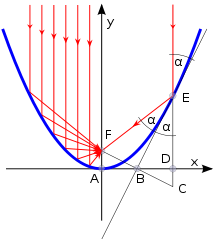Reflective property of a parabola

The reflective property states that, if a parabola can reflect light, then light which enters it travelling parallel to the axis of symmetry is reflected to the focus. This is derived from the wave nature of light in the paragraph "description of final diagram", which describes a diagram just above it, at the end of this article. This derivation is valid, but may not be satisfying to readers who would prefer a mathematical approach. In the following proof, the fact that every point on the parabola is equidistant from the focus and from the directrix is taken as axiomatic.

Consider the parabola y = x2. Since all parabolas are similar, this simple case represents all others. The right-hand side of the diagram shows part of this parabola.

Construction and definitions

The point E is an arbitrary point on the parabola, with coordinates (x, x2). The focus is F, the vertex is A (the origin), and the line FA (the y-axis) is the axis of symmetry. The line EC is parallel to the axis of symmetry, and intersects the x-axis at D. The point C is located on the directrix (which is not shown, to minimize clutter). The point B is the midpoint of the line segment FC.

Deductions

Measured along the axis of symmetry, the vertex, A, is equidistant from the focus, F, and from the directrix. Correspondingly, since C is on the directrix, the y-coordinates of F and C are equal in absolute value and opposite in sign. B is the midpoint of FC, so its y-coordinate is zero, so it lies on the x-axis. Its x-coordinate is half that of E, D, and C, i.e. x/2. The slope of the line BE is the quotient of the lengths of ED and BD, which is x2/x/2, which comes to 2x. But 2x is also the slope (first derivative) of the parabola at E. Therefore, the line BE is the tangent to the parabola at E.

The distances EF and EC are equal because E is on the parabola, F is the focus and C is on the directrix. Therefore, since B is the midpoint of FC, triangles △FEB and △CEB are congruent (three sides), which implies that the angles marked α are congruent. (The angle above E is vertically opposite angle ∠BEC.) This means that a ray of light which enters the parabola and arrives at E travelling parallel to the axis of symmetry will be reflected by the line BE so it travels along the line EF, as shown in red in the diagram (assuming that the lines can somehow reflect light). Since BE is the tangent to the parabola at E, the same reflection will be done by an infinitesimal arc of the parabola at E. Therefore, light that enters the parabola and arrives at E travelling parallel to the axis of symmetry of the parabola is reflected by the parabola toward its focus.

The point E has no special characteristics. This conclusion about reflected light applies to all points on the parabola, as is shown on the left side of the diagram. This is the reflective property.

Other consequences

There are other theorems that can be deduced simply from the above argument.

Tangent bisection property

The above proof, and the accompanying diagram, show that the tangent BE bisects the angle ∠FEC. In other words, the tangent to the parabola at any point bisects the angle between the lines joining the point to the focus, and perpendicularly to the directrix.

Intersection of a tangent and perpendicular from focus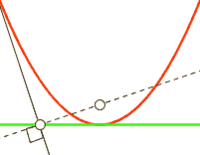Perpendicular from focus to tangent

Since triangles △FBE and △CBE are congruent, FB is perpendicular to the tangent BE. Since B is on the x-axis, which is the tangent to the parabola at its vertex, it follows that the point of intersection between any tangent to a parabola and the perpendicular from the focus to that tangent lies on the line that is tangential to the parabola at its vertex. See animated diagram.

Reflection of light striking the convex side

If light travels along the line CE, it moves parallel to the axis of symmetry and strikes the convex side of the parabola at E. It is clear from the above diagram that this light will be reflected directly away from the focus, along an extension of the segment FE.

Alternative proofs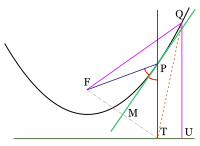Parabola and tangent

The above proofs of the reflective and tangent bisection properties use a line of calculus. For readers who are not comfortable with calculus, the following alternative is presented.

In this diagram, F is the focus of the parabola, and T and U lie on its directrix. P is an arbitrary point on the parabola. PT is perpendicular to the directrix, and the line MP bisects angle ∠FPT. Q is another point on the parabola, with QU perpendicular to the directrix. We know that FP = PT and FQ = QU. Clearly, QT > QU, so QT > FQ. All points on the bisector MP are equidistant from F and T, but Q is closer to F than to T. This means that Q is to the left of MP, i.e. on the same side of it as the focus. The same would be true if Q were located anywhere else on the parabola (except at the point P), so the entire parabola, except the point P, is on the focus side of MP. Therefore, MP is the tangent to the parabola at P. Since it bisects the angle ∠FPT, this proves the tangent bisection property.

The logic of the last paragraph can be applied to modify the above proof of the reflective property. It effectively proves the line BE to be the tangent to the parabola at E if the angles α are equal. The reflective property follows as shown previously.

Tangent properties

Let the line of symmetry intersect the parabola at point Q, and denote the focus as point F and its distance from point Q as f. Let the perpendicular to the line of symmetry, through the focus, intersect the parabola at a point T. Then (1) the distance from F to T is 2f, and (2) a tangent to the parabola at point T intersects the line of symmetry at a 45° angle.:p.26

Orthoptic property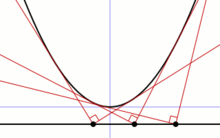Perpendicular tangents intersect on the directrix
Main article: Orthoptic (geometry)

If two tangents to a parabola are perpendicular to each other, then they intersect on the directrix. Conversely, two tangents which intersect on the directrix are perpendicular.

Proof

Without loss of generality, consider the parabola y = ax2, a ≠ 0. Suppose that two tangents contact this parabola at the points (p, ap2) and (q, aq2). Their slopes are 2ap and 2aq respectively. Thus the equation of the first tangent is of the form y = 2apx + C, where C is a constant. In order to make the line pass through (p, ap2), the value of C must be ap2, so the equation of this tangent is y = 2apxap2. Likewise, the equation of the other tangent is y = 2aqxaq2. At the intersection point of the two tangents, 2apxap2 = 2aqxaq2. Thus 2x(pq) = p2q2. Factoring the difference of squares, cancelling, and dividing by 2 gives x = p + q/2. Substituting this into one of the equations of the tangents gives an expression for the y-coordinate of the intersection point: y = 2ap(p + q/2)ap2. Simplifying this gives y = apq.

We now use the fact that these tangents are perpendicular. The product of the slopes of perpendicular lines is −1, assuming that both of the slopes are finite. The slopes of our tangents are 2ap and 2aq, so (2ap)(2aq) = −1, so pq = −1/4a2. Thus the y-coordinate of the intersection point of the tangents is given by y = −1/4a. This is also the equation of the directrix of this parabola, so the two perpendicular tangents intersect on the directrix.

Lambert's theorem

Let three tangents to a parabola form a triangle. Then Lambert's theorem states that the focus of the parabola lies on the circumcircle of the triangle.:Corollary 20

Tsukerman's converse to Lambert's theorem states that, given three lines that bound a triangle, if two of the lines are tangent to a parabola whose focus lies on the circumcircle of the triangle, then the third line is also tangent to the parabola.

Focal length calculated from parameters of a chord

Suppose a chord crosses a parabola perpendicular to its axis of symmetry. Let the length of the chord between the points where it intersects the parabola be c and the distance from the vertex of the parabola to the chord, measured along the axis of symmetry, be d. The focal length, f, of the parabola is given by:Proof

Suppose a system of Cartesian coordinates is used such that the vertex of the parabola is at the origin, and the axis of symmetry is the y-axis. The parabola opens upward. It is shown elsewhere in this article that the equation of the parabola is 4fy = x2, where f is the focal length. At the positive x end of the chord, x = c/2 and y = d. Since this point is on the parabola, these coordinates must satisfy the equation above. Therefore, by substitution, 4fd = (c/2)2
. From this, f = c2/16d.

Area enclosed between a parabola and a chord

Parabola (magenta) and line (lower light blue) including a chord (blue). The area enclosed between them is in pink. The chord itself ends at the points where the line intersects the parabola.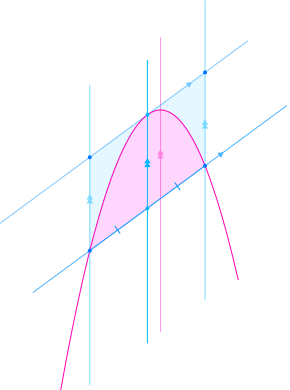The area enclosed between a parabola and a chord (see diagram) is two-thirds of the area of a parallelogram which surrounds it. One side of the parallelogram is the chord, and the opposite side is a tangent to the parabola. The slope of the other parallel sides is irrelevant to the area. Often, as here, they are drawn parallel with the parabola's axis of symmetry, but this is arbitrary.

A theorem equivalent to this one, but different in details, was derived by Archimedes in the 3rd Century BCE. He used the areas of triangles, rather than that of the parallelogram.[lower-alpha 7] See the article "The Quadrature of the Parabola".

If the chord has length b, and is perpendicular to the parabola's axis of symmetry, and if the perpendicular distance from the parabola's vertex to the chord is h, the parallelogram is a rectangle, with sides of b and h. The area, A, of the parabolic segment enclosed by the parabola and the chord is therefore:This formula can be compared with the area of a triangle: 1/2bh.

In general, the enclosed area can be calculated as follows. First, locate the point on the parabola where its slope equals that of the chord. This can be done with calculus, or by using a line that is parallel with the axis of symmetry of the parabola and passes through the midpoint of the chord. The required point is where this line intersects the parabola.[lower-alpha 8] Then, using the formula given in the article "Distance from a point to a line", calculate the perpendicular distance from this point to the chord. Multiply this by the length of the chord to get the area of the parallelogram, then by 2/3 to get the required enclosed area.

Corollary concerning midpoints and endpoints of chords

A corollary of the above discussion is that if a parabola has several parallel chords, their midpoints all lie on a line which is parallel to the axis of symmetry. If tangents to the parabola are drawn through the endpoints of any of these chords, the two tangents intersect on this same line parallel to the axis of symmetry.[lower-alpha 9]

Length of an arc of a parabola

If a point X is located on a parabola which has focal length f, and if p is the perpendicular distance from X to the axis of symmetry of the parabola, then the lengths of arcs of the parabola which terminate at X can be calculated from f and p as follows, assuming they are all expressed in the same units.[lower-alpha 10]This quantity, s, is the length of the arc between X and the vertex of the parabola.

The length of the arc between X and the symmetrically opposite point on the other side of the parabola is 2s.

The perpendicular distance, p, can be given a positive or negative sign to indicate on which side of the axis of symmetry X is situated. Reversing the sign of p reverses the signs of h and s without changing their absolute values. If these quantities are signed, the length of the arc between any two points on the parabola is always shown by the difference between their values of s. The calculation can be simplified by using the properties of logarithms:This can be useful, for example, in calculating the size of the material needed to make a parabolic reflector or parabolic trough.

This calculation can be used for a parabola in any orientation. It is not restricted to the situation where the axis of symmetry is parallel to the y-axis.

Focal length and radius of curvature at the vertex

The focal length of a parabola is half of its radius of curvature at its vertex.

Proof

Consider a point (x, y) on a circle of radius R and with centre at the point (0, R). The circle passes through the origin. If the point is near the origin, the Pythagorean theorem shows that:But if (x, y) is extremely close to the origin, since the x-axis is a tangent to the circle, y is very small compared with x, so y2 is negligible compared with the other terms. Therefore, extremely close to the origin:(Equation 1)

Compare this with the parabola:(Equation 2)

which has its vertex at the origin, opens upward, and has focal length f. (See preceding sections of this article.)

Equations 1 and 2 are equivalent if R = 2f. Therefore, this is the condition for the circle and parabola to coincide at and extremely close to the origin. The radius of curvature at the origin, which is the vertex of the parabola, is twice the focal length.

Corollary

A concave mirror which is a small segment of a sphere behaves approximately like a parabolic mirror, focusing parallel light to a point which is midway between the centre and the surface of the sphere.

Mathematical generalizations

In algebraic geometry, the parabola is generalized by the rational normal curves, which have coordinates (x, x2, x3,…,xn); the standard parabola is the case n =2, and the case n = 3 is known as the twisted cubic. A further generalization is given by the Veronese variety, when there is more than one input variable.

In the theory of quadratic forms, the parabola is the graph of the quadratic form x2 (or other scalings), while the elliptic paraboloid is the graph of the positive-definite quadratic form x2 + y2 (or scalings) and the hyperbolic paraboloid is the graph of the indefinite quadratic form x2y2. Generalizations to more variables yield further such objects.

The curves y = xp for other values of p are traditionally referred to as the higher parabolas, and were originally treated implicitly, in the form xp = kyq for p and q both positive integers, in which form they are seen to be algebraic curves. These correspond to the explicit formula y = xp/q for a positive fractional power of x. Negative fractional powers correspond to the implicit equation xpyq = k, and are traditionally referred to as higher hyperbolas. Analytically, x can also be raised to an irrational power (for positive values of x); the analytic properties are analogous to when x is raised to rational powers, but the resulting curve is no longer algebraic, and cannot be analyzed via algebraic geometry.

Parabolas in the physical world

In nature, approximations of parabolas and paraboloids are found in many diverse situations. The best-known instance of the parabola in the history of physics is the trajectory of a particle or body in motion under the influence of a uniform gravitational field without air resistance (for instance, a ball flying through the air, neglecting air friction).

The parabolic trajectory of projectiles was discovered experimentally by Galileo in the early 17th century, who performed experiments with balls rolling on inclined planes. He also later proved this mathematically in his book Dialogue Concerning Two New Sciences.[lower-alpha 11] For objects extended in space, such as a diver jumping from a diving board, the object itself follows a complex motion as it rotates, but the center of mass of the object nevertheless forms a parabola. As in all cases in the physical world, the trajectory is always an approximation of a parabola. The presence of air resistance, for example, always distorts the shape, although at low speeds, the shape is a good approximation of a parabola. At higher speeds, such as in ballistics, the shape is highly distorted and does not resemble a parabola.

Another hypothetical situation in which parabolas might arise, according to the theories of physics described in the 17th and 18th centuries by Sir Isaac Newton, is in two-body orbits; for example the path of a small planetoid or other object under the influence of the gravitation of the Sun. Parabolic orbits do not occur in nature; simple orbits most commonly resemble hyperbolas or ellipses. The parabolic orbit is the degenerate intermediate case between those two types of ideal orbit. An object following a parabolic orbit would travel at the exact escape velocity of the object it orbits; objects in elliptical or hyperbolic orbits travel at less or greater than escape velocity, respectively. Long-period comets travel close to the Sun's escape velocity while they are moving through the inner solar system, so their paths are close to being parabolic.

Approximations of parabolas are also found in the shape of the main cables on a simple suspension bridge. The curve of the chains of a suspension bridge is always an intermediate curve between a parabola and a catenary, but in practice the curve is generally nearer to a parabola, and in calculations the second degree parabola is used. Under the influence of a uniform load (such as a horizontal suspended deck), the otherwise catenary-shaped cable is deformed toward a parabola. Unlike an inelastic chain, a freely hanging spring of zero unstressed length takes the shape of a parabola. Suspension-bridge cables are, ideally, purely in tension, without having to carry other, e.g. bending, forces. Similarly, the structures of parabolic arches are purely in compression.

Paraboloids arise in several physical situations as well. The best-known instance is the parabolic reflector, which is a mirror or similar reflective device that concentrates light or other forms of electromagnetic radiation to a common focal point, or conversely, collimates light from a point source at the focus into a parallel beam. The principle of the parabolic reflector may have been discovered in the 3rd century BC by the geometer Archimedes, who, according to a legend of debatable veracity, constructed parabolic mirrors to defend Syracuse against the Roman fleet, by concentrating the sun's rays to set fire to the decks of the Roman ships. The principle was applied to telescopes in the 17th century. Today, paraboloid reflectors can be commonly observed throughout much of the world in microwave and satellite-dish receiving and transmitting antennas.

In parabolic microphones, a parabolic reflector that reflects sound, but not necessarily electromagnetic radiation, is used to focus sound onto a microphone, giving it highly directional performance.

Paraboloids are also observed in the surface of a liquid confined to a container and rotated around the central axis. In this case, the centrifugal force causes the liquid to climb the walls of the container, forming a parabolic surface. This is the principle behind the liquid mirror telescope.

Aircraft used to create a weightless state for purposes of experimentation, such as NASA's "Vomit Comet", follow a vertically parabolic trajectory for brief periods in order to trace the course of an object in free fall, which produces the same effect as zero gravity for most purposes.

In the United States, vertical curves in roads are usually parabolic by design.

Click on any image to enlarge it.

Click on any image to enlarge it. To shrink back, return to previous page.

Description of final diagram

Parabolic curve showing chord (L), focus (F), and vertex (V). L is an arbitrary chord of the parabola perpendicular to its axis of symmetry, which passes through V and F. (The ends of the chord are not shown here.) The lengths of all paths QnPnF are the same, equalling the distance between the chord L and the directrix. (See previous image.) This is similar to saying that a parabola is an ellipse, but with one focal point at infinity. It also directly implies, by the wave nature of light, that parallel light arriving along the lines QnPn will be reflected to converge at F. A linear wavefront along L is concentrated, after reflection, to the one point where all parts of it have travelled equal distances and are in phase, namely F. No consideration of angles is required.

Footnotes

1. The tangential plane just touches the conical surface along a line which passes through the apex of the cone
2. Illustrations of these definitions can be found in the gallery of diagrams, below.
3. In the diagram, the axis is not exactly vertical. This is the result of a technical problem that occurs when a 3-dimensional model is converted into a 2-dimensional image. Readers should imagine the cone rotated slightly clockwise, so the axis, AV, is vertical.
4. In the preceding section, the focal length is called p.
5. As stated above in the lead, the focal length of a parabola is the distance between its vertex and focus.
6. The point V is the centre of the smaller circular cross-section of the cone. The point F is in the (pink) plane of the parabola, and the line VF is perpendicular to the plane of the parabola.
7. Archimedes proved that the area of the enclosed parabolic segment was 4/3 as large as that of a triangle that he inscribed within the enclosed segment. It can easily be shown that the parallelogram has twice the area of the triangle, so Archimedes' proof also proves the theorem with the parallelogram.
8. This method can be easily proved correct by calculus. It was also known and used by Archimedes, although he lived nearly 2000 years before calculus was invented.
9. A proof of this sentence can be inferred from the proof of the orthoptic property, above. It is shown there that the tangents to the parabola y = x2 at (p, p2) and (q, q2) intersect at a point whose x-coordinate is the mean of p and q. Thus if there is a chord between these two points, the intersection point of the tangents has the same x-coordinate as the midpoint of the chord.
10. In this calculation, the square root, q, must be positive. The quantity ln a is the natural logarithm of a, i.e. its logarithm to base e.
11. However, this parabolic shape, as Newton recognized, is only an approximation of the actual elliptical shape of the trajectory, and is obtained by assuming that the gravitational force is constant (not pointing toward the center of the earth) in the area of interest. Often, this difference is negligible, and leads to a simpler formula for tracking motion.
1. Wilson, Ray N. (2004). Reflecting Telescope Optics: Basic design theory and its historical development (2 ed.). Springer. p. 3. ISBN 3-540-40106-7. Extract of page 3
2. Stargazer, p. 115.
3. Stargazer, pp. 123 and 132
4. Fitzpatrick, Richard (July 14, 2007). "Spherical Mirrors". Electromagnetism and Optics, lectures. University of Texas at Austin. Paraxial Optics. Retrieved October 5, 2011.
5. Lawrence, J. Dennis, A Catalog of Special Plane Curves, Dover Publ., 1972.
6. Sylvester Reese and Jonathan Sondow. "Universal Parabolic Constant". MathWorld. , a Wolfram Web resource.
7. Tsukerman, Emmanuel (2013). "On Polygons Admitting a Simson Line as Discrete Analogs of Parabolas" (PDF). Forum Geometricorum. 13: 197–208.8. Downs, J. W. (2003). Practical Conic Sections. Dover Publishing.
9. Sondow, Jonathan (2013). "The parbelos, a parabolic analog of the arbelos". American Mathematical Monthly. 120: 929–935. arXiv:1210.2279.
10. Tsukerman, Emmanuel (2014). "Solution of Sondow's problem: a synthetic proof of the tangency property of the parbelos". American Mathematical Monthly. 121: 438–443. arXiv:1210.5580.
11. "Sovrn Container". Mathwarehouse.com. Retrieved 2016-09-30.
12. "Parabola". Mysite.du.edu. Retrieved 2016-09-30.
13. Dialogue Concerning Two New Sciences (1638) (The Motion of Projectiles: Theorem 1); see
14. Troyano, Leonardo Fernández (2003). Bridge engineering: a global perspective. Thomas Telford. p. 536. ISBN 0-7277-3215-3.
15. Drewry, Charles Stewart (1832). A memoir of suspension bridges. Oxford University. p. 159.
16. Middleton, W. E. Knowles (December 1961). "Archimedes, Kircher, Buffon, and the Burning-Mirrors". Isis. Published by: The University of Chicago Press on behalf of The History of Science Society. 52 (4): 533–543. doi:10.1086/349498. JSTOR 228646.
• Lockwood, E. H. (1961). A Book of Curves. Cambridge University Press.Wikimedia Commons has media related to Parabolas.Wikisource has the text of the 1911 Encyclopædia Britannica article Parabola.